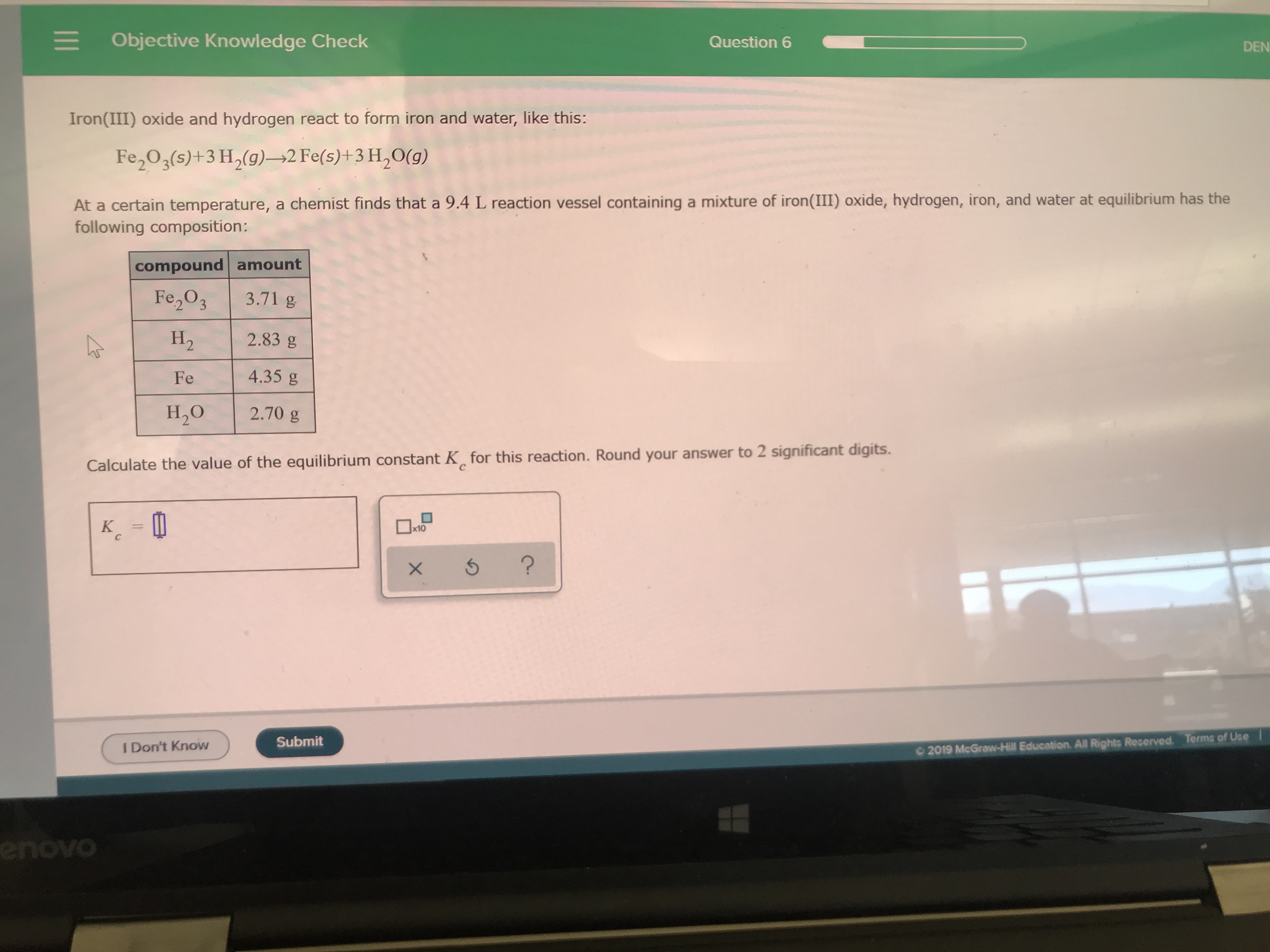# Iron(III) oxide and hydrogen react to form iron and water like this: Fe2O3 (s) + 3 H2(g) 2Fe(s) + 3 H2O (g) At a certain temperature, a chemist finds that a 9.4 L reaction vessel containing a mixture of iron (III) oxide hydrogen iron and water at equilibrium has the following composition. Calculate the value of the equilibrium constant Kc for this reaction